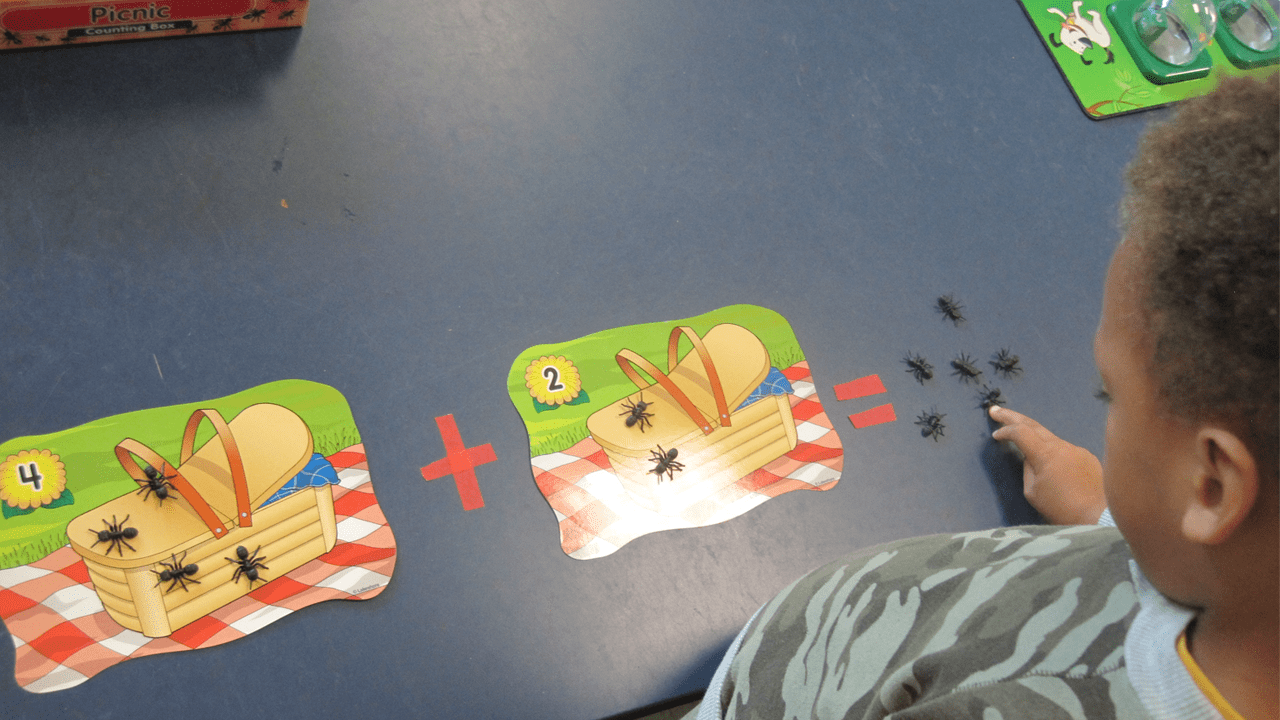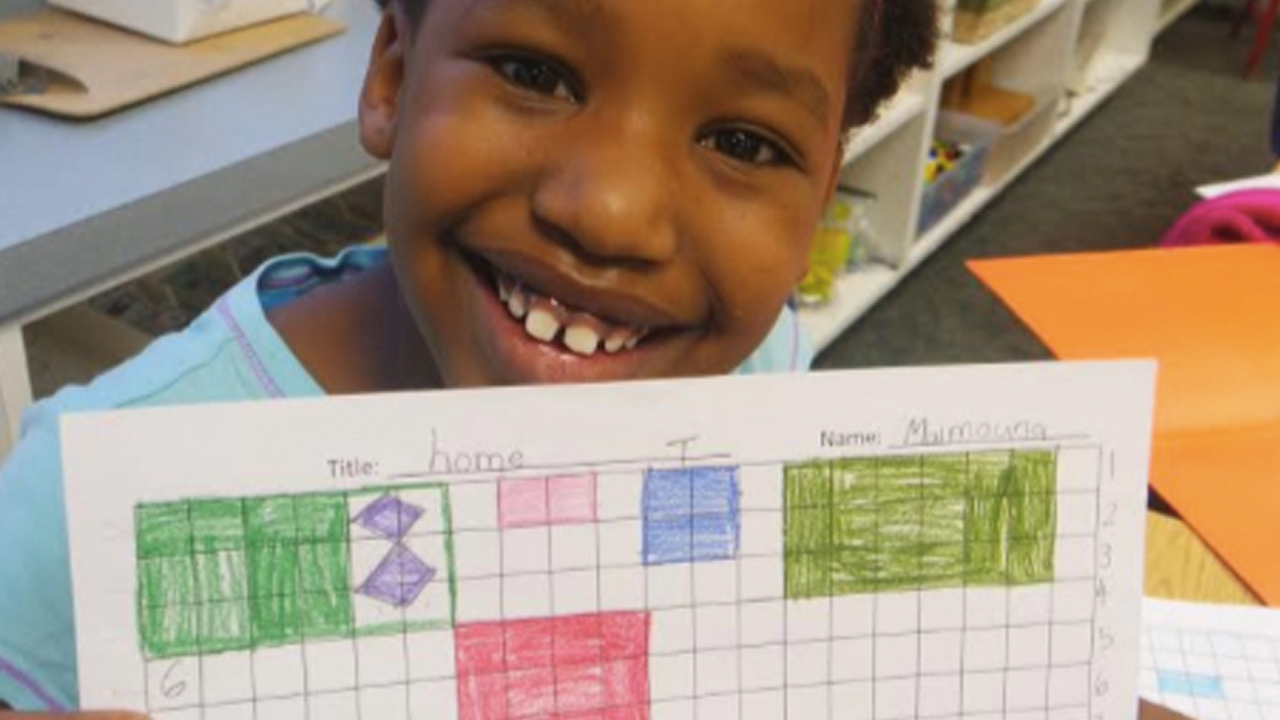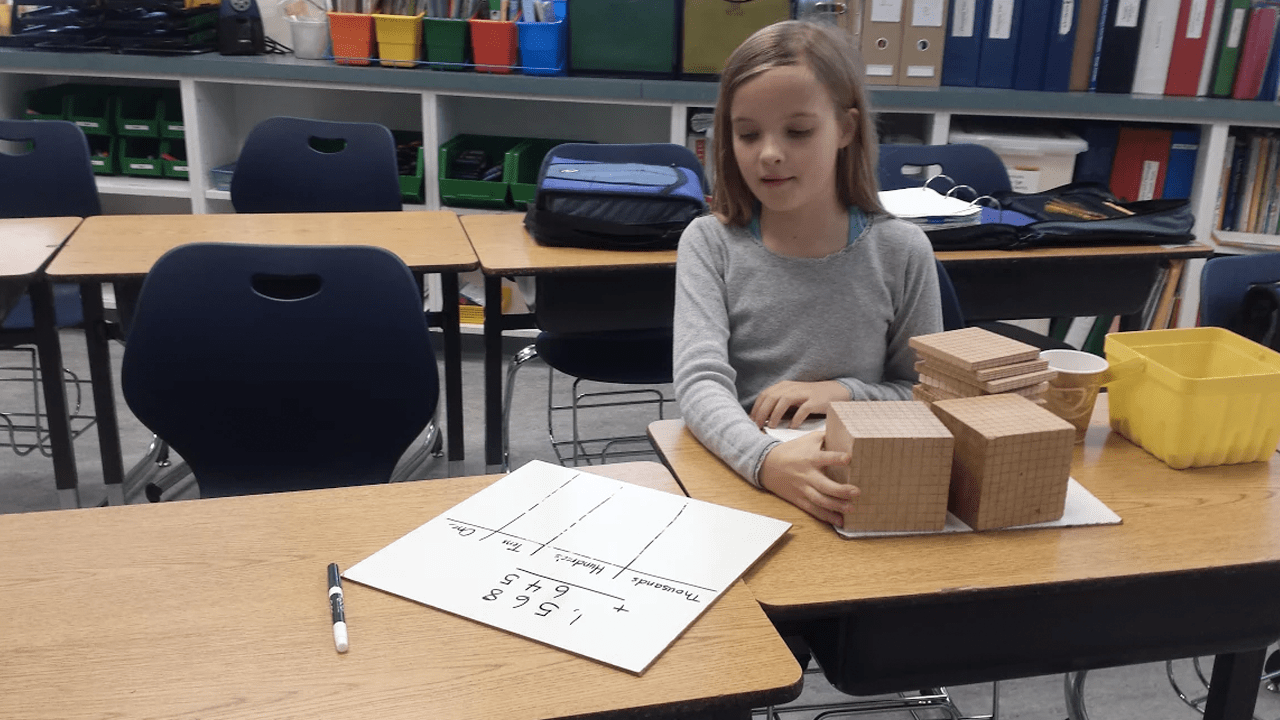•Image 1 of 4
PK/K
•Image 2 of 4
•Image 3 of 4
•Image 4 of 4

# Adding it Up: How Your Lower School Child Gains Addition & Subtraction Skills

Your elementary school math memories may include completing worksheets with rows and rows of addition and subtraction problems.

Since those days that so many of us experienced, a world of research has enlightened educators about how children learn mathematical concepts best. While worksheets still have a place, flexible thinking, problem-solving, number concepts, and having a “growth mindset” have increasingly become more central to developing students’ long-term understanding of and success in math.

Nowhere is the application of this new research more apparent than throughout our Lower School math program.

With increasing complexity, students use multiple ways to explore a single mathematical concept, including manipulatives, math games, roleplay, “number talks,” and verbal and written work (to learn to express themselves accurately). With these approaches, math comes alive, and students develop deeper understandings than through worksheets alone; they support each other’s efforts and learn to persevere using growth mindset techniques.

Addition and subtraction are the foundation of complex mathematical concepts and skills covered across grade levels. Take a look at how we engage your child by in developmentally strengthening ways:

PK/K
The goal of the PK/K math program is to develop an understanding of mathematical concepts through the use of concrete objects and experiential learning, without adding the demand of language. The activities in our PK/K program are designed so students see relationships and interconnections within mathematics through real-life activities and everyday applications

Addition is an abstract concept that we introduce using manipulatives such as unifix cubes, colored beans, manipulative counters, games, and real-world scenarios. Through these activities, our children develop the understanding that addition involves adding two separate groups (addends) to make a new group (sum). The plus and equal symbols are introduced through meaningful teacher-directed and child-centered activities. In these ways, students develop numerical understanding, symbolic understanding, and a positive disposition toward mathematics, all through these addition and subtraction activities.
In first grade, we also teach addition skills in a variety of ways. At morning meeting, children represent the number of the day using tally marks, sticks, base ten blocks, and coins. The students also play games that require adding: Top It, UNO, and Mancala. Finally, the children use the FASTTMATH app on the iPads for fact fluency and are given one-minute quizzes to grasp how quickly they can add simple facts from 0-9.

One way in which students apply their addition and subtraction understanding in second grade is through their imaginary roles as architects and interior designers, planning room layouts and furnishings.

While planning spaces, students need to be aware furnishings cost money. They add costs for sinks, beds, tables, and even entertainment centers. To parallel student learning about socioeconomic status and access, teachers provide a variety of budgets after dream furnishings are selected. Students often subtract selections to rein in the sum of costs to meet different budgets. This authentic application of addition and subtraction in the context of a real-life situation supports the meaningful, long-lasting understanding of addition and subtraction concepts.

In third grade, we challenge students to expand their conceptual understanding of a base-ten system (where place values are ones, tens, hundreds, thousands and so on) by travelling to the “Land of Four” where they learn addition and subtraction through chip trading in a base-four system (where place values are ones, fours, eights, 16s and so on). This learning experience is designed to support student understanding of how a numeric system works, reinforcing place value and the underlying manner in which each digit has a value based on its place in a number.

The “partial sums” algorithm (adding sums one place value at a time) is emphasized to further support place value understanding, and other methods are explored including changing to more “friendly numbers” (rounding numbers up or down by the same amount to make computations easier) and even exploring the “traditional” algorithm. Students are encouraged to practice a variety of addition and subtraction methods through games, and through this work, they discover that no one algorithm is best. In the process, students develop deeper understanding of numbers and the ability to think flexibly with them. These aspects of mathematical thinking are critical to long-term success in higher math processes.

In fourth grade, students expand their exploration of multi-digit addition. In one activity, students challenge each other to read multi-digit numbers correctly, which reinforces place value. Some numbers get incredibly large as they create larger and larger numbers!

Students also apply their skills to find ballpark estimates and solve addition number stories. They estimate sums to project the amount of food they consume in a year and present their findings with verbal explanations of their work. Requiring students to estimate sums reinforces numeric understanding. Using a map, students also calculate estimated distances between American cities they would like to visit and the amount of time required to drive from one city to another. In addition to working with whole numbers, students work on adding multi-digit decimals as well as fractions. When diving into this arena of number exploration, students’ prior understanding of the base-ten system and place value serves a critical role.

In fifth grade we continue to build on a student’s knowledge of addition facts by adding decimals, fractions, and mixed numbers; solving number stories; writing open number sentences, making magnitude estimates, and creating circle graphs.

Name That Number is a favorite fifth grade game where students strive to create on-target numbers using a set of five Everyday Math Deck cards. Students then add, subtract, multiply, and/or divide using as many of their five cards as possible to equal the target number. This game provides a great opportunity for students to talk about the strategies they use to mentally find an answer while using different mathematical operations. Such discussions also require students to explain their thinking–a key component of what “real” mathematicians do. This explanation also forces students to use their reasoning and logical thinking skills to communicate with others.

Content for this post provided by:

Laura Yee, LS principal
Susie Loutoo, 4th grade teacher and LS math department chair
Jessica Townsend, PK/K teacher
Joann Phinney, K teacher
Skyle Pearson, 1st grade teacher
Danny Stock, 2nd grade teacher
Holly Balshem, 3rd grade math instructor
Jessica Ahn, 3rd grade teacher
Bryan Williams, 5th grade math teacher
Luisa Myavec, 5th grade math teacher